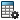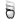Assessing Force Contributions to Reflected Load Inertia or Mass

The contribution of external or internal forces to the reflected load inertia or mass calculation is additive. You can calculate the contribution of forces, such as gravity, friction, or external forces to reflected load inertia or mass by defining a reflected load inertia or reflected load mass result and calculating the motion with and without the force in effect.

To assess the contribution of gravity to reflected load inertia from a Motion Analysis type study for a model with a rotary motor:

1. Define a reflected load inertia result for the rotary motor.
2. Apply a gravity force to the model.
3. Click Calculate(MotionManager toolbar).
The reflected load inertia plot appears.
4. Right-click the result plot, click Export CSV, and save the results to a .csv file.
5. Right-click Gravityin the MotionManager tree and click Suppress.
6. Repeat Steps 3. and 4, exporting the result to another .csv file.
7. Compare the exported results.